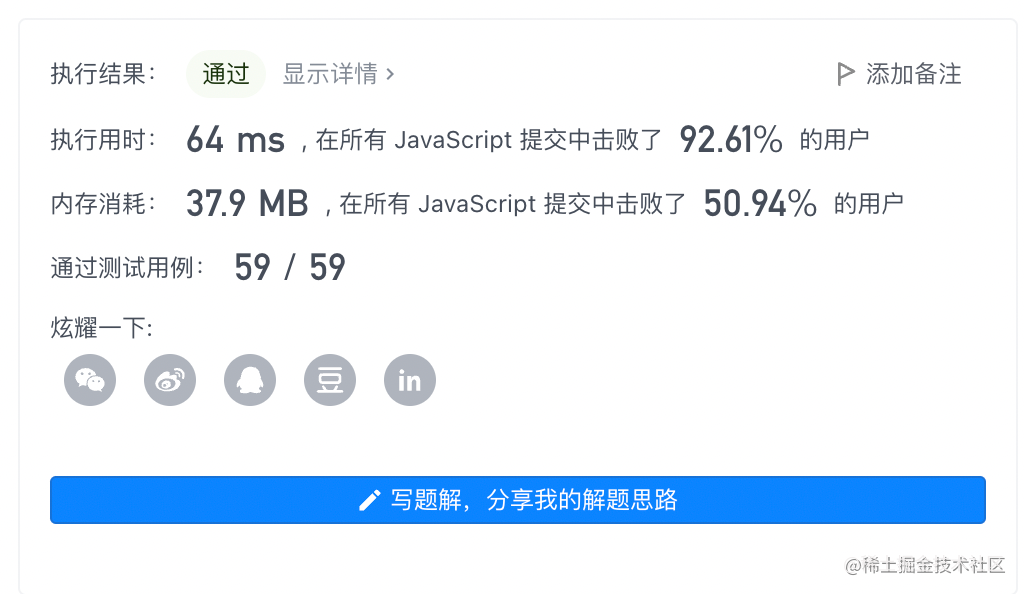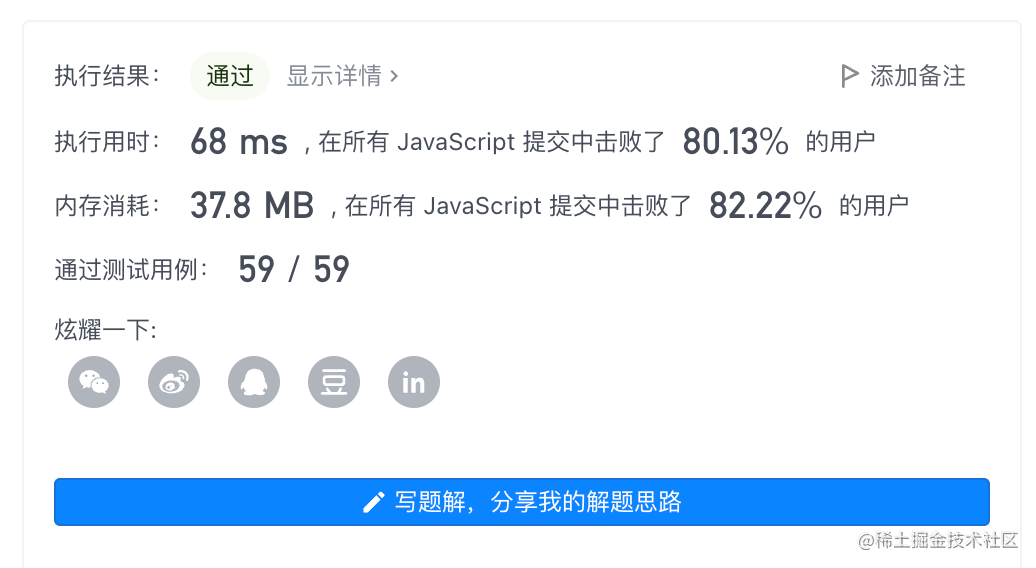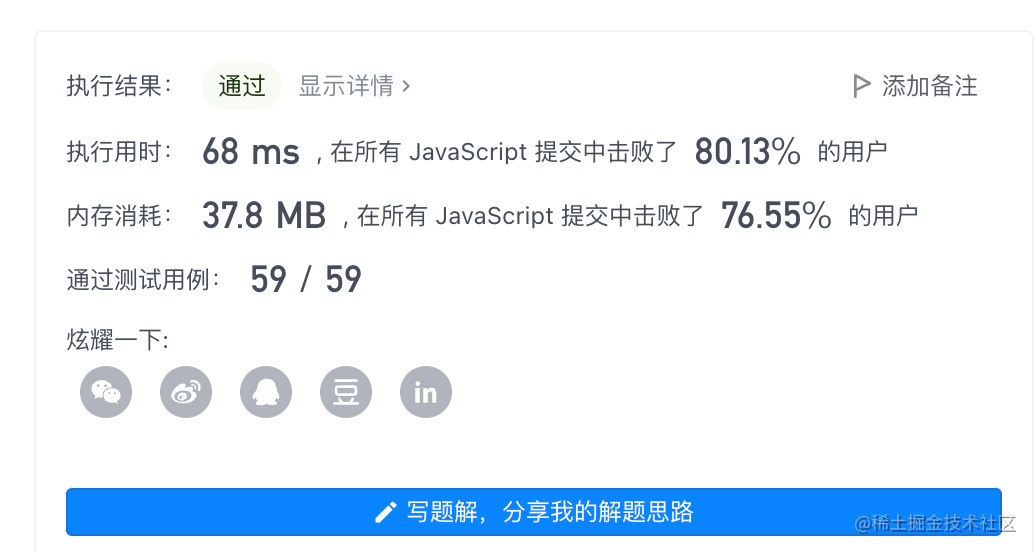2022-11-1700

## 目录

1. 题目
2.解法一：直接合并后排序
3.解法二：双指针法
4.解法三：双指针优化（逆向双指针）
5.总结

# 1. 题目

• 示例 1：
``````输入：nums1 = [1,2,3,0,0,0], m = 3, nums2 = [2,5,6], n = 3

``````
• 示例 2：
``````输入：nums1 = , m = 1, nums2 = [], n = 0

``````
• 示例 3：
``````输入：nums1 = , m = 0, nums2 = , n = 1

``````

# 2.解法一：直接合并后排序

```javascript```var merge = function(nums1, m, nums2, n) {
nums1.splice(m, nums1.length - m, ...nums2);
nums1.sort((a, b) => a - b);
};
``````• 复杂度分析：
• 时间复杂度：不同浏览器对sort的实现不同，这里按快速排序算，平均情况为`O((m+n)log(m+n))`

• 空间复杂度：`log(m + n)`

# 3.解法二：双指针法

```javascript```/**
* @param {number[]} nums1
* @param {number} m
* @param {number[]} nums2
* @param {number} n
* @return {void} Do not return anything, modify nums1 in-place instead.
*/
var merge = function(nums1, m, nums2, n) {
let result = []
let nums1Index = nums2Index = 0

while(nums1Index < m && nums2Index < n) {
if(nums1[nums1Index] <= nums2[nums2Index]) {
result[nums1Index + nums2Index] = nums1[nums1Index++]
} else {
result[nums1Index + nums2Index] = nums2[nums2Index++]
}
}

while(nums1Index < m) {
result[nums1Index + nums2Index] = nums1[nums1Index++]
}

while(nums2Index < n) {
result[nums1Index + nums2Index] = nums2[nums2Index++]
}

const length = result.length
for(let i = 0; i < length; i++) {
nums1[i] = result[i]
}
};
``````
• 复杂度分析：
• 时间复杂度：** `O(m+n)`** 因为最多只用遍历一边num1和num2两个数组

• 空间复杂度：`O(m+n)` 需要建立长度为 m+n 的中间数组# 4.解法三：双指针优化（逆向双指针）

```javascript```/**
* @param {number[]} nums1
* @param {number} m
* @param {number[]} nums2
* @param {number} n
* @return {void} Do not return anything, modify nums1 in-place instead.
*/
var merge = function(nums1, m, nums2, n) {

let nums1Index = m - 1
let nums2Index = n - 1
while(nums1Index >=0 && nums2Index >= 0) {
if(nums1[nums1Index] >= nums2[nums2Index]) {
nums1[nums1Index + nums2Index + 1] = nums1[nums1Index--]
} else {
nums1[nums1Index + nums2Index + 1] = nums2[nums2Index--]
}
}

while(nums2Index >= 0) {
nums1[nums2Index] = nums2[nums2Index--]
}
};
``````
• 复杂度分析：
• 时间复杂度： `O(m+n)` 因为最多只用遍历一边num1和num2两个数组

• 空间复杂度：`O(1)` 直接对数组 `nums1` 原地修改，不需要额外空间。# 5.总结

`从结果看上去好像解法一更快，但还是要从复杂度分析上来看`

• 解法一： 时间复杂度`O((m+n)log(m+n))` 空间复杂度`log(m + n)`
• 解法二： 时间复杂度`O(m+n)` 空间复杂度`O(m+n)`
• 解法三： 时间复杂度`O(m+n)` 空间复杂度`O(1)` 因此解法三更优

`ps：但是平时在开发中数据量不太会影响效率的时候，我会用第一种，因为实在太简洁了😁`